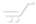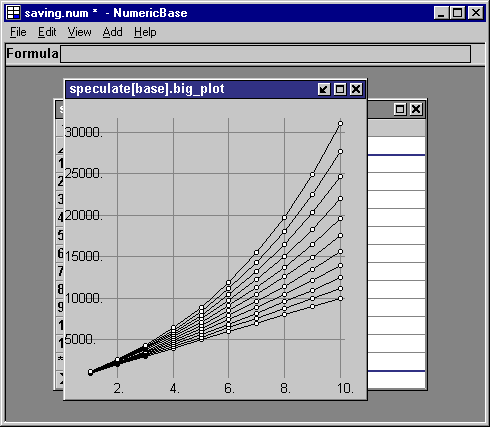# Saving account scenarios

Different interest rates.
This example shows what will happen to our saving account from the previous example, under different interest rate. Interest rate are ranging from 0 to 20 - we want to see the end balance in each case, and a plot of the balance.

The solution is based on the last solution with the addition of another table. The table have the following columns:
• interest : has the formula =range 0 to 20 . Responsible to generate the range of different interest rates
• tables : has the formula: =Table2(intrest:=interest).   this formula instantiates the table from the previous example, and replace the interest rate with a different interest rate for each row.
• big_plot : has the formula =collect(tables.p) - collects the balance plot from each instantiated table to display the balances under different interest-rate scenarios in one plot.
• end_balance collects the end balance of each scenarios to be displayed.Screenshot: different interest rate scenarios.

You can see the a chart showing the balances under different interest rate scenarios, by clicking on the "multi plot" icon in the previous screenshot. This chart is featured in the next screenshot.balances under different interest rate scenarios

You can also see a table showing the balances for a specific scenario, by clicking on of the table icon at column " tables " , the following screenshot shows the table for interest rate=0.Screenshot: table for interest rate=0

The source code
Following is the source code for this example. To use it in your copy of NumericBase, copy and paste it in the View Source window.

table Table2{//based on the previous example   balance:=(prev+investment)*(1+interest/100)!   investment:=1000!   year:=range 1 to term!   interest:=10!   term:=10!   p:=plot(year,balance)! }   table speculate{//adding the interest-rate scenario   interest:=range 0 to 20 step 2!   tables:=Table2(interest:=interest)!   big_plot:=collect(tables.p)!   end_balance:=tables.balance! } optimized(60,60) result(speculate); optimized(32,44) result(speculate.tables);
Listing: source code of the Saving account scenarios example